﻿ Application of the Triangular Fuzzy Model to Assessment of Analogical Reasoning Skills

### Application of the Triangular Fuzzy Model to Assessment of Analogical Reasoning Skills

Michael Gr. Voskoglou, Igor Ya. SubbotinOPEN ACCESSPEER-REVIEWED

## Application of the Triangular Fuzzy Model to Assessment of Analogical Reasoning Skills

Michael Gr. Voskoglou1,, Igor Ya. Subbotin2

1School of Technological Applications, Graduate Technological Educational Institute (T. E. I.) of Western Greece, Patras, Greece

2College of Letters and Sciences, National University of Los Angeles, California, USA

### Abstract

We apply the Triangular Fuzzy Assessment Model (TFAM) for analogical reasoning skills assessment. The TFAM is a new original model based on the Centre of Gravity (COG) defuzzification technique, which we have properly adapted and used in earlier papers as an assessment method of several human activities. The main idea of the TFAM is the replacement of the rectangles appearing in the graph of the COG technique by isosceles triangles sharing common parts. In this way, we can treat better the ambiguous cases of scores being at the boundaries between two successive linguistic characterizations (grades) of the level of the individuals’ performance. An application is also presented illustrating our results in practice, where the TFAM is compared with two traditional assessment methods (calculation of the means and of the GPA index) based on principles of the bivalent logic (yes-no).

### At a glance: Figures

1
Prev Next

• Voskoglou, Michael Gr., and Igor Ya. Subbotin. "Application of the Triangular Fuzzy Model to Assessment of Analogical Reasoning Skills." American Journal of Applied Mathematics and Statistics 3.1 (2015): 1-6.
• Voskoglou, M. G. , & Subbotin, I. Y. (2015). Application of the Triangular Fuzzy Model to Assessment of Analogical Reasoning Skills. American Journal of Applied Mathematics and Statistics, 3(1), 1-6.
• Voskoglou, Michael Gr., and Igor Ya. Subbotin. "Application of the Triangular Fuzzy Model to Assessment of Analogical Reasoning Skills." American Journal of Applied Mathematics and Statistics 3, no. 1 (2015): 1-6.

 Import into BibTeX Import into EndNote Import into RefMan Import into RefWorks

### 1. Introduction

In 1999 Voskoglou developed a fuzzy model for the description of the learning process [15, 18] and later  he used the total uncertainty of the corresponding fuzzy system for assessing the students’ skills in learning mathematics. Meanwhile Subbotin et al. , based on Voskoglou’s  fuzzy model, adopted properly the widely used in Fuzzy Mathematics Center of Gravity (COG) defuzzification technique to provide an alternative measure for the assessment of students’ learning skills. Since then, both Voskoglou and Subbotin, either collaborating or independently to each other, utilized the COG technique in assessing other students’ competencies (e.g. see [11, 14, 20, 21], etc) as well as the Bridge player’s performance  and for testing the effectiveness of a CBR system .

The first attempt to apply the Triangular Fuzzy Assessment Model (TFAM) was made in , while more recently Subbotin and Voskoglou  presented an improved version of it for assessing students’ critical thinking skills. The main idea of the TFAM is to replace the rectangles appearing in the membership function’s graph of our COG assessment model by triangles sharing common parts, which must be considered twice in calculating the COG of the level’s area lying between the resulting graph and the OX axis. In this way, one succeeds to treat better the ambiguous cases of students’ scores being at the boundaries between two successive grades. It is a very common approach in such cases to divide the interval of the specific grades in three parts and to assign the corresponding grade using + and -. For example, in a scale of scores from 0 to 100 we could have 75 – 77 = B-, 78 – 80 = B, 81 – 84 = B+. However, this consideration does not reflect the common situation, where the teacher is not sure about the grading of the students whose performance could be assessed as marginal between and close to two adjacent grades; for example, something like between 74 (C) and 75 (B). The TFAM fits to this kind of situations.

This paper aims at using an extended version of the TFAM for obtaining a fuzzy measure of students’ Analogical Reasoning (AR) skills. The rest of the paper is organized as follows: In section 2 we describe the AR process. In section 3 we develop the TFAM using a scale of five grades (A, B, C, D and F) instead of the three grades (A, B, C-F) used in  and . In this way the assessment method obtained becomes more accurate. In section 4 we present a classroom application performed recently with students of the Graduate Technological Educational Institute (T. E. I.) of Western Greece illustrating our results in practice. Finally, section 5 is devoted to conclusions and discussion about our plans for further research on the subject.

For general facts on fuzzy sets we refer to the book .

### 2. Analogical Reasoning

Reasoning is the most important human brain operation. In particular, much of our cognitive activity does depend on our ability to reason analogically. Analogies are used for explaining concepts which cannot directly perceived (e.g. electricity in terms of the water flow), in making predictions within domains, in communication and persuasion, etc.

AR is actually a method of processing information that compares the similarities between new and past understood concepts, then using these similarities to gain understanding of the new concept.

AR is important in general for creativity and scientific discovery. Within cognitive science mental processes are likened to computer programs (e.g. neural networks) and such analogies serve as mental models to support reasoning in new domains. In Artificial Intelligence the Case-Based-Reasoning paradigm covers a range of different methods (including AR) for organizing, retrieving, utilizing and indexing the knowledge of past cases [19, 23].

Solution of problems by analogy (analogical problem solving) is probably the most important aspect of AR. However this strategy can be difficult to implement in problem solving, because it requires the solver to attend to information other than the problem to be solved (usually referred as the target problem). Thus the solver may come up empty-handed, either because he/she has not solved any similar problems in past, or because he/she fails to realize the relevance of previous problems. But, even if an analogue is retrieved, the solver must know how to use it to determine the solution procedure for the target problem.

A characteristic example is the experiment of Gick and Holyoak [3, 4] on a problem known as the Dunker’s tumor problem: You have a patient with an inoperable stomach tumor. There are some rays that, at sufficient intensity, destroy organic tissue. How can you free the patient of the tumor without destroying the healthy tissue surrounding it?

The desired solution is to use a system of multiple machines to emit low-intensity rays from different directions. These rays will converge on the tumor and their combined effect will destroy it.

In the first phase of the experiment only a 10% of the subjects gave the correct solution. Next, before presenting the problem to another group of subjects, an analogous story was given to read about a general, who wants to capture a fortress and he is able to do so by sending parts of his army down each of several roads, all of which converge on the fortress. In this case the percentage of the correct solutions increased to the 30% of the subjects, while a further spectacular increase to 70% happened when subjects were given the hint to use the story above for the solution of the target problem.

Several studies (e.g. [1, 2, 6, 8, 16, 23], etc) have provided detailed models for the process of AR, which are broadly consistent with reviews of problem solving strategy training studies, in which factors associated with instances of successful transfer – that is, use of already existing knowledge to produce new knowledge - are identified. According to these studies the main steps of the AR process include:

•  Representation of the target problem.

•  Search-retrieval of a related past problem.

•  Mapping of the representations of the target and the related problem.

•  Adaptation of the solution of the related problem for use with the target problem.

More specifically, before solvers working on a problem they usually construct a representation of it. A good representation must include both the surface and the structural (abstract, solution relevant) features of the problem. The former are mainly determined by what are the quantities involved in the problem and the latter by how these quantities are related to each other. The features included in solvers' representations of the target problem are used as retrieval cues for a related problem in memory, usually referred as a source problem. When the two problems share structural but not surface features the source is called a remote analogue of the target problem.

Analogical mapping requires aligning the two situations – that is, finding the correspondences between the representations of the target and the source problem – and projecting inferences from the source to the target problem. Once the common alignment and the candidate inferences have been discovered, the analogy is evaluated. The last step involves the adaptation of the solution of the analogous problem for use with the target problem, where the correspondences between objects and relations of the two problems must be used.

The successful completion of the AR process is referred as positive analogical transfer. But the search may also yield distracting problems having surface but not structural (solution relevant) common features with the target problem and therefore being only superficially similar to it. The reason for this is usually a non satisfactory representation of the target problem, containing only its salient surface features, and the resulting consequences on the retrieval cues available for the search process. When a distracting problem is considered as an analogue of the target, we speak about negative analogical transfer. This happens when a distracting problem is retrieved as a source problem and the solver fails, through the mapping of the representations of the source and target problem, to realize that the source cannot be considered as an analogue to the target. Therefore the process of mapping is very important in AR playing the role of a "control system" for the fitness of the source problem.

### 3. The Triangular Fuzzy Assessment Model

Let U= {A, B, C, D, F} be the set of students’ grades A= excellent, B = very good, C = good, D = fair and F = not satisfactory. In applying the COG as an assessment method, we represent the student group under assessment as a fuzzy set in U and we associate to each x in U an interval of the OX axis (i.e. we replace U with a set of real intervals). Then, we construct the graph of the corresponding membership function, which, in this particular case, is a bar graph consisting of five rectangles with one side lying on the OX axis (e.g. see Figure 1 of ). Next, using the well known from Mechanics formulas, we calculate the coordinates (Xc, Yc) of the COG of the level’s section lying between this graph and the OX axis, thus obtaining the defuzzification of the corresponding fuzzy data. Further, using elementary algebraic inequalities we determine the area where the COG lies and by elementary geometric observations we obtain a criterion about the student group’s performance (for details see, for example, section 2 of ).

As said in our introduction, for applying the TFAM instead of the classical COG technique, we replace the rectangles appearing in the graph of the COG technique by triangles. Therefore, we shall have five such triangles in the resulting scheme, each one corresponding to a students’ grade (F, D, C, B and A respectively). Without loss of generality and for making our calculations easier we consider isosceles triangles with bases of length 10 units lying on the OX axis. The height to the base of each triangle is equal to the percentage of students’ of the group under assessment who achieved the corresponding grade. We allow for any two adjacent triangles to have 30% of their bases belonging to both of them. In this way, we cover the situation of uncertainty in assessing marginal students’ scores, as we have described in our introduction.

The resulting scheme is presented in Figure 1. The student group under assessment can be represented again, as in the COG technique, as a fuzzy set in U, whose membership function y = m(x) has as graph the line OA1B1A2B2A3 B3A4 B4A5C9. It is easy then to calculate the coordinates (bi1, bi2) of the points Bi, i = 1, 2, 3, 4, 5. In fact, B1 is the intersection of the straight line segments A1C2 and C1A2, B2 is the intersection of C3A3, and A2C4 and so on. Therefore, it is straightforward to determine the analytic form of y=m(x) consisting of 10 branches, corresponding to the equations of the straight lines OA1, A1B1, B1A2, A2B2, B2A3, A3B3, B3A4, A4B4, B4A5 and A5C9 in the intervals [0, 5), [5, b11), [b11, 12), [12, b12), [b12, 19), [19, b13), [b13, 26), [26. b14), [b14, 33) and [33, 38] respectively.

However, when applying the TFAM, the use of the analytic form of y = m(x) is not needed (in contrast to the case of the classical COG technique) for the calculation of the COG of the resulting level’s area. In fact, since the marginal cases of students’ grades should be considered as common parts for any pair of the adjacent triangles, it is logical to don’t subtract the areas of the intersections from the area of the corresponding level’s section, although in this way we count them twice; e.g. placing the ambiguous cases B+ and A- in both regions B and A. In other words, the classical COG technique, which calculates the coordinates of the COG of the area between the graph of the membership function (line OA1B1A2B2A3 B3A4 B4A5C9) and the OX axis (see Figure 1), thus considering the areas of the “common” triangles C1B1C2, C3B2C4, C5B3C6 and C7B4C8 only once, is not the proper one to be applied in the above situation.

Indeed, in this case it is reasonable to represent each one of the five triangles OA1C2, C1A2C4, C3A3C6, C5A4C8 and C7A5C9 of Figure 1 by their COG’s Fi, i=1, 2, 3, 4, 5 and to consider the entire level’s area as the system of these points-centers. More explicitly, the steps of the whole construction of the TFAM are the following:

1. Let y1, y2, y3, y4, y5 be the percentages of the students in the group getting F, D, C, B, and A grades respectively, then y1 + y2 + y3 + y4 + y5 = 1 (100%).

2. We consider the isosceles triangles with bases having lengths of 10 units each and their heights y1, y2, y3, y4, y5 in the way that has been illustrated in Figure 1. Each pair of adjacent triangles has common parts in the base with length 3 units.

3. We calculate the coordinates () of the COG Fi, i=1, 2, 3, 4, 5 of each triangle as follows: The COG of a triangle is the point of intersection of its medians, and since this point divides the median in proportion 2:1 from the vertex, we find, taking also into account that the triangles are isosceles, that. Further, since the triangles’ bases have a length of 10 units, it is easy to observe that xci = 7i-2.

4. We consider the system of the centers Fi, i=1, 2, 3, 4, 5 and we calculate the coordinates (Xc, Yc) of the COG Fc of the whole level’s area considered in Figure 1 from the following formulas, derived from the commonly used in such cases definition (e.g. see ):(1)

In formulas (1) S denotes the whole of the considered level’s area and Si, i= 1, 2, 3, 4, 5 denote the areas of the corresponding triangles. Therefore Si = 5yi and S=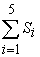=5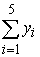=5. Thus, from formulas (1) we finally get:(2)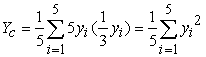5. We determine the area where the COG Fc lies as follows: For i, j=1, 2, 3, 4, 5, we have that

0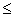(yi –yj)2=yi2+yj2-2yiyj, therefore yi2+yj2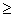2yiyj, with the equality holding if, and only if, yi=yj. Therefore(3)

with the equality holding if, and only if, y1 = y2 = y3 = y4 = y5 =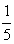. In the case of equality the first of formulas (2) gives that Xc = 7(+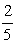+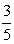+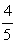+) –2 = 19. Further, combining the inequality (3) with the second of formulas (2) one finds that Yc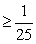Therefore the unique minimum for Yc corresponds to the COG Fm (19,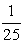). The ideal case is when y1=y2=y3= y4=0 and y5=1. Then from formulas (2) we get that Xc = 33 and Yc =.Therefore the COG in this case is the point Fi (33,). On the other hand, the worst case is when y1=1 and y2= y3 = y4= y5=0. Then from formulas (2), we find that the COG is the point Fw (5,). Therefore the “area” where the COG Fc lies is the triangle Fw Fm Fi presented in Figure 2.

6. We formulate our criterion for comparing the performance of two (or more) groups’ as follows: From elementary geometric observations (see Figure 2) it follows that for two groups the group having the greater Xc performs better. Further, if the two groups have the same Xc ≥19, then the group having the COG which is situated closer to Fi is the group with the greater Yc. Also, if the two groups have the same Xc<19, then the group having the COG which is situated farther to Fw is the group with the smaller Yc. Based on the above considerations it is logical to formulate our criterion for comparing the two groups’ performance in the following form:

•  Among two or more groups the group with the greater Xc performs better.

•  If two or more groups have the same Xc 19, then the group with the greater Yc performs better.

•  If two or more groups have the same Xc < 19, then the group with the lower Yc performs better.

### 4. An Application to AR

In order to illustrate the use of the TFAM as an assessment method of AR skills we performed the following experiment, in which the subjects were students of the Graduate Technological Educational Institute of Western Greece, in the city of Patras. We formed two groups, say G1 and G2, of 34 and 51 students respectively from two different Departments of the School of Technological Applications (prospective engineers) being at their first term of studies.

Two mathematical problems were given for solution to both groups (see Appendix). The first of them was on combinatorial analysis and probability and the second was on the theory of matrices, which are topics of the students’ first term course of mathematics. In each case and before receiving the target problem students received three other problems together with their solution procedures. They read each problem and its solution procedure and then solved in detail the problem themselves using the given procedure. Students were allowed 10 minutes for each problem and they were not given the other problem until after 10 minutes had elapsed. The first of these problems was a remote analogue of the target problem, the second was a distracting problem, while the third was unrelated to the target problem. Next the target problem was given and it was asked to students to try to solve it by adapting the solution of one of the previous three problems (time allowed 20 minutes).

The students’ solutions of the target problems were marked in a scale from 0 to 100 and their performance was characterized as follows: A (excellent) = 85-100, B (very good) = 75-84, C (good) = 60-74, D (fair) = 50-59 and F (not satisfactory) = 0-49

The scores achieved by the students of the two groups were the following:

First group (G1): 100(1 time), 98(1), 95(3), 94(2), 93(1), 88(1), 85(3), 82(4), 80(3), 76(1), 74(2), 73(1), 63(1), 59(2), 58(1), 55(2), 52(1), 48(1), 45(2), 38(1).

Second group (G2): 99(1), 95(3), 88(3), 85(5), 82(3), 80(2), 78(4), 75(9), 70(2), 64(3), 60(4), 58(2), 56(3), 50(4), 45(1), 40(1), 26(1).

The above data is summarized in the following Table:

The evaluation of this data will be performed below in two ways: i) By using two traditional assessment methods based on principles of the bivalent logic (yes-no) and ii) by applying the TFAM. The results obtained will be discussed and compared to each other.

a) Calculation of the means: Adding all students’ scores (presented above) for each group and dividing the corresponding sum by the total number of students of the group (34 for G1 and 51 for G2) one finds that the means of the students’ scores are approximately 74.97 and 71.24 for the first and the second group respectively. This shows that the mean performance of both groups was good, with the performance of the first group being better.

b) Calculation of the GPA index: We recall that the Great Point Average (GPA) index is a weighted mean, where more importance is given to the higher scores, to which greater coefficients (weights) are attached (e.g. see ). In other words, the GPA index focuses on the quality performance of a student group.

In fact, let us denote by nA, nB, nC, nD and nE the numbers of students whose performance was characterized by A, B, C, D and F respectively and by n the total number of students of each group.. Then the GPA index is calculated by the formula GPA=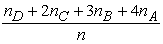. In terms of the notation used in section 3 the above formula can be written in the form GPA = y2+2y3+3y4+4y5 (4).

In the ideal case (y1 = y2 = y3 = y4 = 0, y5 = 1) the equation (4) gives that GPA = 4, while in the worst case (y1 = 1, y2 = y3 = y4 = y5 = 0) it gives that GPA = 0. Therefore we have that 0GPA4.

In our case, from Table 1 one finds that the percentages of the students in each group getting the grades F, D, C, B, and A respectively are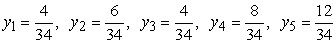for G1 and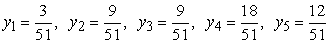for G2. Therefore (4) gives that GPA==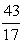2.529 for G1. Similarly one finds that the GPA index takes the same value for G2. Thus, since the above value of the GPA index is greater than the half of its maximal value, which is equal to 4, the two student groups demonstrated a satisfactory quality performance.

4.2. Application of the TFAM

From the description of the TFAM presented in section 3 it becomes evident that in this case a group’s performance is actually measured by the abscissa Xc of the COG. Thus, observing the first of formulas (2) it is easy to realize that the TFAM, similarly with the GPA index, measures the group’s quality performance.

In the case of our experiment, applying the first of formulas (2) on the data of Table 1 it is straightforward to check that the value of Xc for both groups is equal to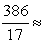22.71 > fact, according to the students’ percentages (see calculation of the GPA index in section 4.1) we find for G1 that Xc = 7(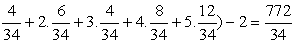and a similar argument holds for G2. Thus, since the value 22.71 is much greater than the half of the value 33 of Xc in the ideal case, both groups demonstrated a good quality performance.

Further, from the second of formulas (2) one finds that Yc =0.048 for G1 and similarly Yc =0.049 for G2. Therefore according to our criterion stated in section 3, the performance of the second group was slightly better.

4.3. Comparison of the Results of the Assessment Methods

The application of the above - three in total - methods for assessing students’ AR skills resulted to different conclusions. In fact, in the first case (calculation of the means) G1 demonstrated a better performance than G2, while according to the GPA index the two groups demonstrated equal performances. However, according to the TFAM, G2 demonstrated a better performance than G1!

The above conclusions are not embarrassing at all, since, in contrast to the calculation of the means, which focuses on a group’s mean performance, the GPA and the TFAM methods focus on its quality performance by assigning greater coefficients to the higher scores. Further, on comparing the first of equations (2) with equation (4), it turns out that the TFAM assigns greater weight coefficients to the higher scores than the GPA index (see Table 2). In other words, TFAM is more sensitive to the higher scores than the GPA index.

#### Table 2. Weight coefficients of the yi’s

In concluding, it is suggested to the user of the above methods to choose the one that it fits better to its personal criteria of goals. However, a combined application of them could help him/her to get a more comprehensive view of the students’ performance.

### 5. Conclusions and Discussion

The common experience shows that the assessment methods of a group’s performance usually applied in practice are those based on principles of the bivalent logic (yes-no). However, fuzzy logic, due to its nature of characterizing the ambiguous cases with multiple values, offers a wider and richer field of resources for this purpose. This gave us the impulsion to introduce in this paper an extended (with 5 grades) version of the TFAM approach for assessing students’ AR skills. TFAM is a recently developed (in a version with 3 grades) variation of the COG defuzzification technique fitting better to the ambiguous cases of students’ scores lying at the boundaries between two different student grades.

However, there is a need for more applications to be performed for obtaining safer statistical data. On the other hand, since the TFAM approach looks to have the potential of a general assessment method, our future plans for further research on the subject include also the effort to apply this approach in assessing the individuals’ performance in several other human activities.

### References

  Gentner, D., Toupin, C., “Systematicity and surface similarity in development of analogy”, Cognitive Science, 10, 277-300, 1986.In article CrossRef  Gentner, D., Markman, A. B., “Structure mapping in analogy and similarity”, American Psychologist 52, 45-56, 1997.In article CrossRef  Gick, M. L., Holyoak, K. J., “Analogical problem solving”, Cognitive Psychology, 12, 306-355, 1980.In article CrossRef  Gick, M. L., Holyoak, K. J., “Schema induction and analogical transfer”, Cognitive Psychology, 15, 1-38, 1983.In article CrossRef  “Grade Point Average Assessment”, available in the Web at: http://www.swinburne.edu.au/student-administration/assessment/gpa.html, visited October 15, 2014.In article  Holyoak K. J., “The pragmatics of analogical transfer”. In G. H. Bower (Ed.), The psychology of learning and motivation, Vol. 19, 59-87, New York: Academic Press., 1985In article  Klir, G. J. & Folger, T. A.., Fuzzy Sets, Uncertainty and Information, Prentice-Hall, London, 1988.In article  Novick, L. R., “Analogical transfer, problem similarity and expertise”, Journal of Educational Psychology: Learning, Memory and Cognition, 14, 510-520, 1988.In article  Subbotin, I. Ya., Badkoobehi, H., Bilotckii, N. N., “Application of fuzzy logic to learning assessment”, Didactics of Mathematics: Problems and Investigations, 22, 38-41, 2004.In article  Subbotin, I. Ya., Voskoglou, M. Gr., “Applications of fuzzy logic to Case-Based Reasoning”, International Journal of Applications of Fuzzy Sets and Artificial Intelligence, 1, 7-18, 2011.In article  Subbotin, I., Mossovar-Rahmani, F., Bilotskii, N., “Fuzzy logic and the concept of the Zone of Proximate Development”, Contemporary trends in the development of mathematics and its application aspects (Proceedings of the 1st International Scientific Internet-Conference), pp. 301-302, Donetsk, 2012.In article  Subbotin, I.Ya., Bilotskii, N. N., “Triangular fuzzy logic model for learning assessment”, Didactics of Mathematics: Problems and Investigations, 41, 84-88, 2014.In article  Subbotin, I. Ya., Voskoglou, M. Gr., “Language, mathematics and critical thinking: The cross influence and cross enrichment”, Didactics of Mathematics: Problems and Investigations, 41 89-94, 2014.In article  Subbotin, I. Ya., Voskoglou M. Gr., “A Triangular Fuzzy Model for Assessing Students’ Critical Thinking Skills”, International Journal of Applications of Fuzzy Sets and Artificial Intelligence, 4, 173-186, 2014.In article  Voskoglou, M. Gr., “The process of learning mathematics: A fuzzy set approach”, Heuristic and Didactics of Exact Sciences,, 10, 9-13, 1999.In article  Voskoglou, M. Gr., “Analogical Problem Solving and Transfer”, Proceedings of the 3d Mediterranean Conference on Mathematical Education, 295-303, Athens, 2003.In article  Voskoglou, M. Gr., “Transition across levels in the process of learning: A fuzzy model”, International Journal of Modelling and Application, Univ. Blumenau, Brazil, 1, 37-44, 2009.In article  Voskoglou, M. Gr., Stochastic and fuzzy models in Mathematics Education, Artificial Intelligence and Management, Lambert Academic Publishing, Saarbrucken, Germany, 2011.In article  Voskoglou, M. Gr., “Case-Based Reasoning: History, Methodology and Development Trends”, International Journal of Psychology Research, 6 (5), 505-530, Nova Publishers, N. Y., 2011.In article  Voskoglou, M. Gr, “Fuzzy measures for students’ mathematical modelling skills”, International Journal of Fuzzy Logic Systems, 2 (2), 13-26, 2012.In article CrossRef  Voskoglou, M. Gr., Subbotin, I. Ya., “Fuzzy models for analogical reasoning”, International Journal of Applications of Fuzzy Sets and Artificial Intelligence, 2, 19-38, 2012.In article  Voskoglou, M. Gr., “Assessing the Players’ Performance in the Game of Bridge: A fuzzy Logic Approach”, American Journal of Applied Mathematics and Statistics, 2 (3), 115-120, 2014.In article CrossRef  Voskoglou, M. Gr., Salem, A.-B. M., “Analogy-Based and Case-Based Reasoning: Two Sides of the Same Coin”, International Journal of Applications of Fuzzy Sets and Artificial Intelligence, 4, 5-51, 2014.In article  Wikipedia, “Center of mass: A system of particles”, available in the Web at: http://en.wikipedia.org/wiki/Center_of_mass#A_system_of_particles, visited on October 10, 2014.In article

### Appendix

List of problems of our classroom experiment and their solutions’ procedure

CASE 1

Target problem: A box contains 8 balls numbered from 1 to 8. One makes three successive drawings, putting back the corresponding ball to the box before the next drawing. Find the probability of getting all the balls drawing out of the box different to each other.

Solution’s sketch: The probability is equal to the quotient of the total number of the ordered samples of 3 objects from 8 (favourable outcomes) to the total number of the corresponding samples with replacement (possible outcomes).

Remote analogue: How many numbers of 2 digits can be formed by using the digits from 1 to 6 and how many of them have their digits different?

Solution’s procedure given to the students: Find the total number of the ordered samples of 2 objects from 6 with and without replacement respectively.

Distracting problem: A box contains 3 white, 4 blue and 6 black balls. If we draw out 2 balls, what is the probability to be of the same colour?

Solution’s procedure given to the students: The number of all favourable outcomes is equal to the sum of the total number of combinations of 3, 4 and 6 objects taken 2 at each time respectively, while the number of all possible outcomes is equal to the total number of combinations of 13 objects taken 2 at each time.

Unrelated problem: Find the number of all possible anagrammatisms of the word “SIMPLE”. How many of them start with S and how many of them start with S and end with E?

Solution’s procedure given to the students: The number of all possible anagrammatisms is equal to the total number 6! of the permutations of 6 objects. The anagrammatisms starting with S are 5! and the anagrammatisms starting with S and ending with E are 4!

CASE 2

Target problem: Consider the matrices: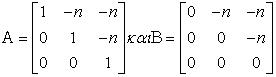Show that An = Α + (n-1)(Β +Β), for every positive integer n.

Solution’s sketch: Since Α=Ι+Β, where I stands for the unitary 3Χ3 matrix, and B3 =0, is Αn=(Ι+Β)n=Ι+nΒ+ +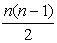Β2 ==Α+(n -1)Β+Β = Α+(n-1)(Β+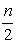Β).

Remote analogue: Let α be a nonzero real number. Prove that αn =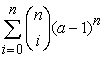, for all positive integers n.

Solution’s procedure given to the students: Write α = 1+(α-1) and apply the Newton’s formula (x+b)n =, setting x=1 and b=α-1.

Distracting problem: If A and B are as in the target problem, calculate (A+B)2.

Solution’s procedure given to the students: Make the corresponding calculations.

Unrelated problem: Prove that 1+2+…+n =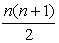, for all positive integers n.

Solution’s procedure given to the students: Apply induction on n.